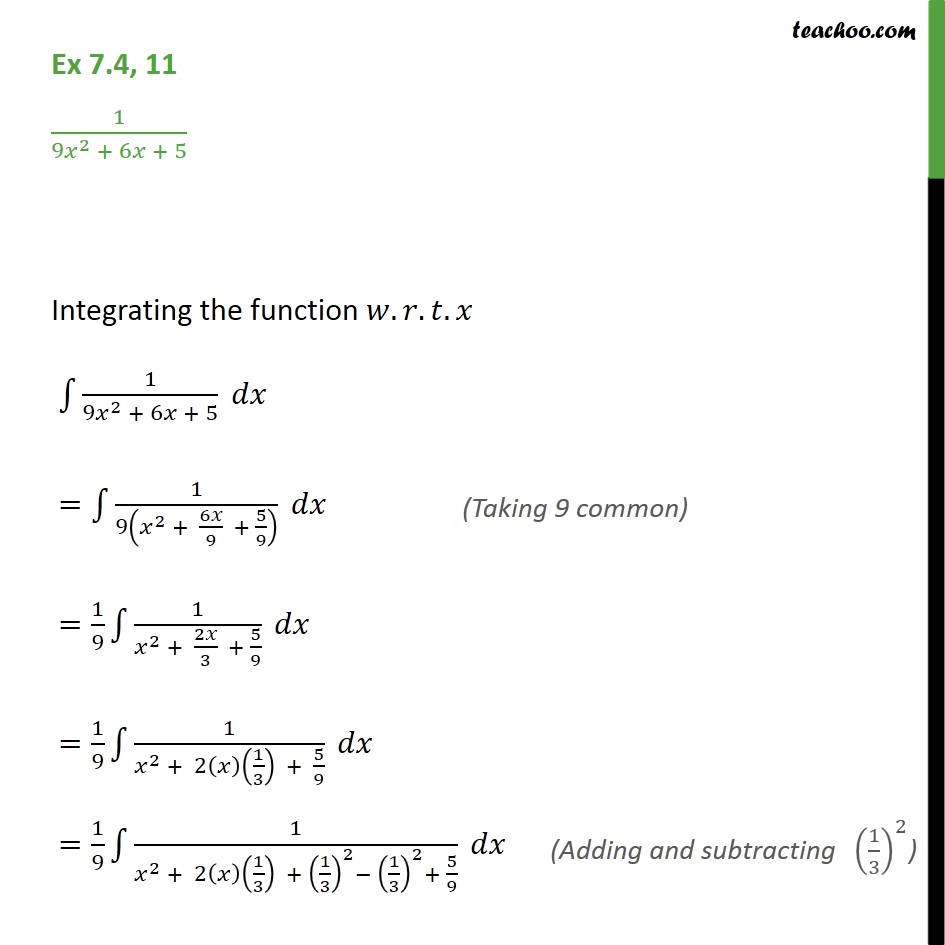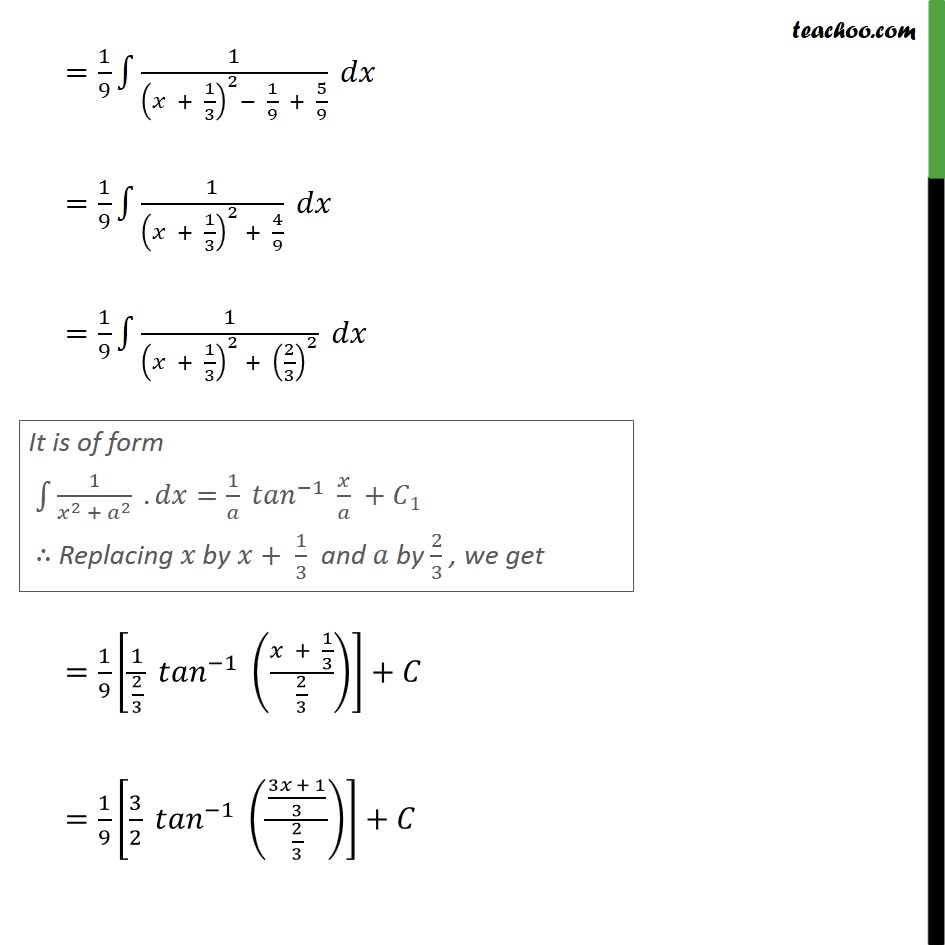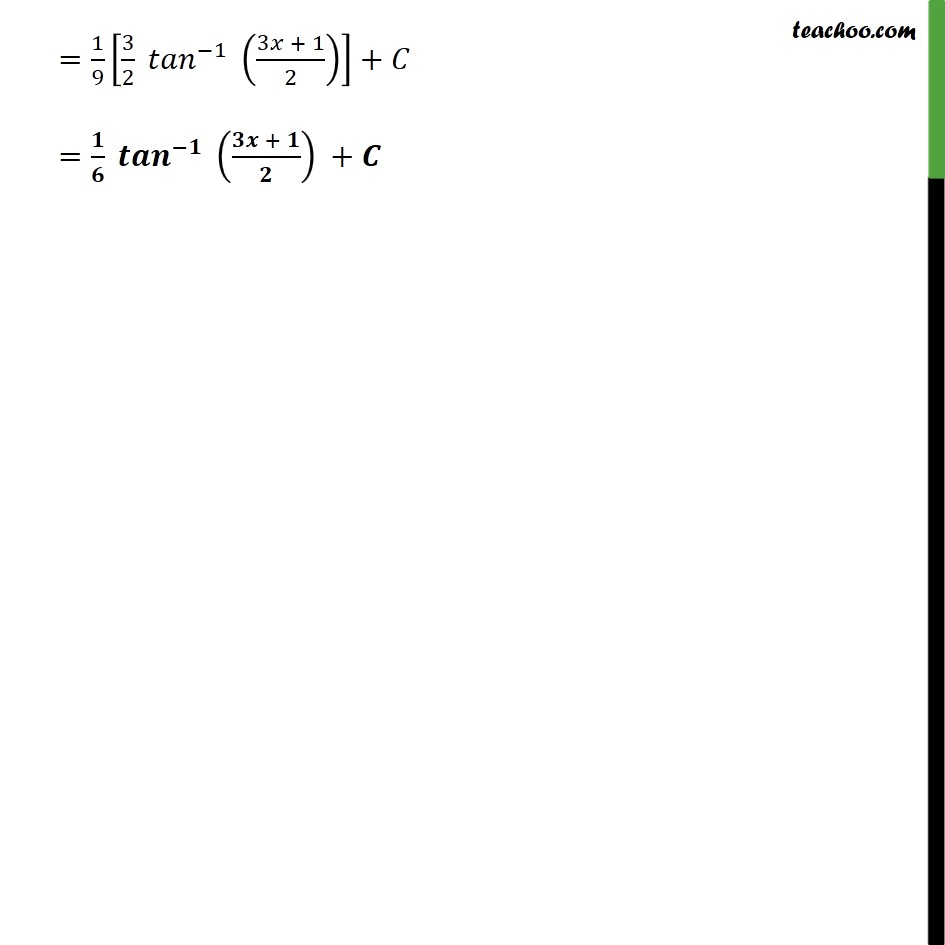1. Chapter 7 Class 12 Integrals (Term 2)
2. Serial order wise
3. Ex 7.4

Transcript

Ex 7.4, 11 1 9 2 + 6 + 5 Integrating the function . . . 1 9 2 + 6 + 5 = 1 9 2 + 6 9 + 5 9 = 1 9 1 2 + 2 3 + 5 9 = 1 9 1 2 + 2 1 3 + 5 9 = 1 9 1 2 + 2 1 3 + 1 3 2 1 3 2 + 5 9 = 1 9 1 + 1 3 2 1 9 + 5 9 = 1 9 1 + 1 3 2 + 4 9 = 1 9 1 + 1 3 2 + 2 3 2 = 1 9 1 2 3 1 + 1 3 2 3 + = 1 9 3 2 1 3 + 1 3 2 3 + = 1 9 3 2 1 3 + 1 2 + = + +

Ex 7.4